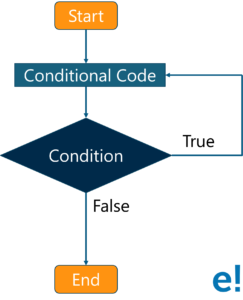# What is a Do while loop in Java and how to use it?

Published on Jul 31,2019 819 Views4 / 5 Blog from Control Statements

The Java do-while loop is used to iterate a set of statements until the given condition is satisfied. If the number of iteration is not fixed and you MUST have to execute the loop at least once, then use a do while loop. In this tutorial, you will learn all about do while loop in Java and how to use it.

Let us dive into the article and explore the following topics:

Let’s begin!

## What is Do while loop in Java?

Do-while loop is similar to while loop, but it possesses one dissimilarity: In while loop, the condition is evaluated before the execution of loop’s body but in do-while loop condition is evaluated after the execution of loop’s body.

I will make the concept visually clear through this flow diagram.

## Flow diagram:Let me explain the above-shown diagram:

1. First of all, it will execute a set of statements that are mentioned in your ‘do’ block.
2. After that, it will come to ‘while’ part where it checks the condition.
3. If the condition is true, it goes back and executes the statements.
4. If the condition is false, it directly exits the loop.

Now, moving on towards the syntax of do while loop in Java

### Syntax:

do{

//code to be executed

}while(condition);

As you can see, the syntax is pretty easy. Next, let me show you the implementation of the do while loop through an example.

## Implementation through example:

``` public class Example {

public static void main(String args[]) {
int x = 1;

do {
System.out.print("value of x : " + x );
x++;
System.out.print("n");
}while( x < 11 );
}
}
```

Output:

value of x : 1
value of x : 2
value of x : 3
value of x : 4
value of x : 5
value of x : 6
value of x : 7
value of x : 8
value of x : 9
value of x : 10

In the above-mentioned code, you can see that the do while loop will execute the condition once, for sure.

Now, let’s hop onto our next segment, Infinite do-while loop in Java.

## Infinite do-while loop in Java

Infinite do-while loop in Java is similar to the infinite while loop. You can refer a section of my previous blog –Infinite while Loop in Java.

If you pass ‘true’ in the do-while loop, it will be infinitive do-while loop. Here is the syntax:

### Syntax:

do{

//code to be executed

}while(true);

Below is a simple example depicting the usage of java infinitive do while loop.

### Example of infinitive do while loop:

```	public class DoWhileInfinite {
public static void main(String[] args) {
do{
System.out.println("infinitive do while loop");
}while(true);
}
}
```

Output:

infinitive do while loop
infinitive do while loop
infinitive do while loop

In order to exit the loop, press ctrl+c.

With this, I have reached towards the end of my blog. I hope the data added value to your Java knowledge. We will continue digging into more concepts of the Java world. Stay tuned!

Check out the Java training by Edureka, a trusted online learning company with a network of more than 250,000 satisfied learners spread across the globe. Edureka’s Java J2EE and SOA training and certification course is designed for students and professionals who want to be a Java Developer. The course is designed to give you a head start into Java programming and train you for both core and advanced Java concepts along with various Java frameworks like Hibernate & Spring.

Got a question for us? Please mention it in the comments section of this ‘’Do while loop in Java” blog and we will get back to you as soon as possible.REGISTER FOR FREE WEBINARThank you for registering Join Edureka Meetup community for 100+ Free Webinars each month